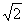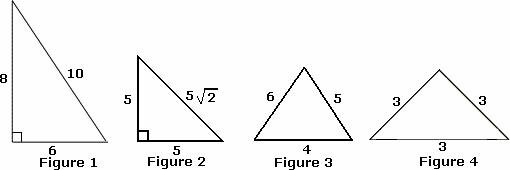Definition Of Isosceles Right Triangle

A right triangle in which two sides and two angles are equal is called Isosceles Right Triangle.

In an isosceles right triangle, the angles are 45°, 45°, and 90°.
Isosceles right triangle satisfies the Pythagorean Theorem.
The length of the hypotenuse in an isosceles right triangle istimes the side's length.

Example of Isosceles Right TriangleThe given figure is an isosceles right triangle as the sides AB and BC are equal in length and angle B is a right angle.

Solved Example on Isosceles Right Triangle

Ques: Which of the following is an isosceles right triangle?A. Figure 3
B. Figure 1
C. Figure 4
D. Figure 2